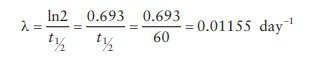| Home | | Pharmaceutical Drugs and Dosage | | Pharmaceutical Industrial Management |

## Chapter: Pharmaceutical Drugs and Dosage: Radiopharmaceuticals

Calculations for radioactive materials are important for dispensing or administering the right dose to a patient has also for the laboratory researcher to use the right amount of the radioactive material.

Calculations

Calculations for radioactive materials are important for dispensing or administering the right dose to a patient has also for the laboratory researcher to use the right amount of the radioactive material. In addi-tion, the phenomenon of radioactive decay necessitates an understanding of changing radioactivity of a preparation over a period of time.

N(t ) = N ( 0 )e−λt

Note that this is a typical first-order equation, where a measure of the rate of a phenomenon [N(t)/N(0)] is an exponential function of the rate of that phenomenon, λ, times the elapsed time. The minus in the exponent repre-sents the reduction of radioactivity with time.

Moreover, since,

λ=1/τ

N(t ) = N ( 0 )et/τ

Thus, radioactive decay is exponential with a constant probability.

Typical of the first-order reaction kinetics, at half-life, t = t1 2 andor, the rate of decay, λ, can be calculated from t1/2 as:As seen in this equation, highly radioactive materials (high λ) have a short half-life, whereas low-radioactivity emitting materials last longer.

Example

Ten mCi of a radioactive isotope was received in the laboratory on February 15. This isotope has a half-life of 60 days. How much is the remaining radioactivity on March 15?

Solution:

N(0) = 10 mCi

t = 30 days

t1/2 = 60 days

N(t) = ?

Now, N(t ) = N(0)e−λt

To use this equation, we need to calculate λ, the rate of decay.Now,

N(t ) = N(0)e − λt = 10 mCi × e 0.01155 ×30 = 7.07 mCi

Note that the initial radioactivity of 10 mCi would become 5 mCi in 60 days, since the t1/2 is 60 days. However, after 30 days of storage, on day 30, the activity of the radioactive compound is more than a quarter of the initial radioactivity. This highlights that the rate of decay of a radioactive compound follows a nonlinear first-order exponential rate kinetics.